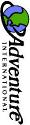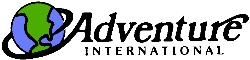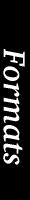Scott Free Gfx Format# An attempt of an explaination By Andreas Aumayr \$VER: 1.01, 29/Feb/2000

## Contents:

1. Introduction
2. Hunk structure
1. Info hunk
2. Offset hunk
3. Logic hunk
4. Data hunk
3. Action 89
4. Tools
5. Conclusion

## Introduction

This gfx format is the result of a sometimes lengthy discussion between Paul David Doherty and myself. Our aim was to create a simple but nevertheless flexible structure with all the graphics information put into one single file.

Certainly we completely failed in our mission but we have an independent "Scott-Free-Gfx-File-Format" now and at least it's just a single file :-)

## Hunk structure

There are 4 hunks and these are:

1. Info hunk
2. Offset hunk
3. Logic hunk
4. Data hunk

### Info hunk

Has just the right complexity for an easy beginning. It only includes information about the picture size = width * height and number of pictures.

```    //Check if gfx are available
gfx_in_file = fopen(gfx_file,"rb");
if (gfx_in_file == NULL) GFX = FALSE;

//Fetch GFX info from binary file (if GFX == TRUE :-))
gfx_width = fgetc(gfx_in_file)*256 + fgetc(gfx_in_file);
gfx_height = fgetc(gfx_in_file)*256 + fgetc(gfx_in_file);

nroom = fgetc(gfx_in_file); // Number of rooms (+1 if showPIC
// starts with 0 and not 1 !!!)
nac89 = fgetc(gfx_in_file); // Number of Action89 pics
fgetc(gfx_in_file);         // Number of extended (conditional) room pics
// (rooms can have more than 1 pic)
```

### Offset hunk

Includes byte offsets from byte 0 of the gfx file to each of the pic data (pic data is in hunk d). Each offset is 3 bytes long.

If a room has no picture, offset is set to zero (Scott Free Amiga closes the picture window in those rare cases).

If there is absolutely nothing to do, offset is 255. This is my prefered case :-).

If two or more different rooms share the same pic, offset is the same, too (thus file length is a bit shorter).

First offset (offset for pic 0) is always the offset to the darkness-pic.

```    count = fgetc(gfx_in_file);                  // number of offsets
// = number of pics
go = malloc(sizeof(unsigned long)  * count); // allocate mem
// for offsets
for (i=0; i<count; i++) {                    // insert offsets into
// offset table
go[i] = (unsigned long) in_dat[i*3]*256*256 +
(unsigned long) in_dat[i*3+1]*256 + in_dat[i*3+2];
}
if (go == 0) LDP = TRUE; 		 // use darkness pic offset
else LDP = FALSE;				 // as LDP Flag
```

### Logic hunk

This hunk basically features the logic when (under which condition or event) to display what picture.

If all of the conditions are false, display the default picture.

Let's show that with the simple example from Adventureland:

Room Picture Condition = Object (Number)
1 (A) present = cypress tree (5)
(B) present = hollow stump (4)
5 (A) present = locked door (16)
(B) present = open door (17)
18 (A) present = stream of lava (34)
(B) present = lava stream with dam (45)
20 (A) present = bricked up window (32)
(B) present = bricked up window with hole (35)
21 (A) present = very thin bear (25)
(B) not present (25)
23 (A) present = large sleeping dragon (27)
(B) not present (27)

That means for Room 1 that there are 2 pictures (picture A and picture B). Display A if the cypress tree (object No. 5) is present (= default picture), display B if the hollow stump (object No. 4) is present, instead. Simple, isn't it?

To put this information into the logic hunk, the following codes are used:

#### Condition codes (CC):

 01 in room 02 in inventory 04 in room or inventory 81 not in room 82 not in inventory 84 not in room nor in inventory

#### Display codes (DC):

 01 unconditional display (followed by picture number) 41 special effect: color cycling 42 special effect: overlay 43 special effect: animation 44 special effect: GO_TREE for "Robin of Sherwood" 81 conditional display, one condition (followed by condition and picture number) 82 conditional display, two conditions (followed by condition 1, picture 1 and condition 2, picture 2) 83 see 81 and 82 ...

That results in this byte order for our example room 1:

 RO NC DC CC ON PN 01 01 81 01 04 38
• RO = room number
• NC = number of conditional pics for room RO
• DC = display code(s)
• CC = condition code(s)
• ON = object number(s)
• PN = picture number(s) to display if condition is true

The sample source to get the logic information:

``` count = fgetc(gfx_in_file)*256
+ fgetc(gfx_in_file);              // how many BYTES
// for room logic
while (j<count) {
rnum = in_dat[j++];                     // room number
rp[rnum].ppr = in_dat[j++];             // number of cond. pics for room
for (i=0; i<rp[rnum].ppr; i++) {
rp[rnum].what[i] = in_dat[j++];      // display code
kk = rp[rnum].what[i]-80;
while (kk < 0) kk += 10;
for (k=0; k<kk; k++) {
rp[rnum].loc[i][k] = in_dat[j++]; // condition code
rp[rnum].obj[i][k] = in_dat[j++]; // object
}
rp[rnum].pnr[i] = in_dat[j++];       // picture number to display
// if condition is TRUE
}
}
```

And here's the source fragment to evaluate the complete logic info:

``` if (rp[Room].ppr) {
for (j=0; j<rp[Room].ppr; j++) {
ii = rp[Room].what[j]-80;
while (ii < 0) ii += 10;
yes = 0;
for (i=0; i<ii; i++) {
switch (rp[Room].loc[j][i]) {
case 1: // in room
if (Items[rp[Room].obj[j][i]].Location == MyLoc) yes++;
break;
case 2: // in inventory
if (Items[rp[Room].obj[j][i]].Location == 255) yes++;
break;
case 4: // in room or inventory
if ((Items[rp[Room].obj[j][i]].Location == 255) ||
(Items[rp[Room].obj[j][i]].Location == MyLoc)) yes++;
break;
case 81: // NOT in room
if (Items[rp[Room].obj[j][i]].Location != MyLoc) yes++;
break;
case 82: // NOT in inventory
if (Items[rp[Room].obj[j][i]].Location != 255) yes++;
break;
case 84: // NOT in room and NOT in inventory
if ((Items[rp[Room].obj[j][i]].Location != 255) &&
(Items[rp[Room].obj[j][i]].Location != MyLoc)) yes++;
break;
default:
break;
}
}
if (yes >= ii) {
if (rp[Room].pnr[j] == 0) {                // no picture
if (pic_w_hdl) {
win_pos_ver = pic_w_hdl->LeftEdge;   // save window position
win_pos_hor = pic_w_hdl->TopEdge;
CloseWindow(pic_w_hdl);
pic_w_hdl = 0;
}
last_pic = -128;                        // remember: no last pic
return(FALSE);
}
if (rp[Room].pnr[j] == 255) return(FALSE); // 255 = do nothing
if (last_pic >= 0) {
if ((go[last_pic] == go[rp[Room].pnr[j]])) return(FALSE);
}
switch ((int)(rp[Room].what[j]/10)) {
case PIC: // Show Pic
Open_Pic(rp[Room].pnr[j],PIC);
return(TRUE);
case COC: // colour cycling, not implemented yet
break;
case OVL: // overlay picture
yes = 1;
if (last_pic != Room) {
for (i=0; i<rp[Room].ppr; i++) {
if (last_pic == rp[Room].pnr[i]) {
yes = 0;
break;
}
}
}
else yes = 0;
if (yes) Open_Pic(Room,PIC); // basis pic is not already displayed
Open_Pic(rp[Room].pnr[j],OVL);
return(TRUE);
case ANI: // animation, not implemented yet
break;
case GOT: // GO_TREE for "Robin of Sherwood", not implemented yet
break;
default:
break;
}
}
}
}
Open_Pic(Room,PIC);
```

### Data hunk

Finally some picture data.

2 Formats are supported: Bitmaps and LineDrawPictures (LDP).

#### Bitmaps

Pictures are stored as chunky pixels, 6 bits per colour (bit 8 is the "repeat-flag" and bit 7 is the "overlay-flag"). Compressing is done via a slightly modified RLE algorithm (not very efficient at all but fast and most important, very easy to implement).

One more word to bit 7 (overlay): If set, keep colour of default pic, else use colour from overlay pic.

Use the source below for reading and decrompressing picture data:

``` fseek(gfx_in_file,go[pic_nr],SEEK_SET);     // set position in file
NumColours = fgetc(gfx_in_file);            // how many colours
for (i=0; i<NumColours; i++) {              // fetch colours in RGB format
CR[i].red = fgetc(gfx_in_file);
CR[i].green = fgetc(gfx_in_file);
CR[i].blue = fgetc(gfx_in_file);
}
fread(&rle_dat,1,size,gfx_in_file);      // get chunky data in rle_dat[]
j = i = 0;                                  // and decompress it
while (j<size) {                            // into picture[]
while (rle_dat[j] < 128) {
picture[i++] = rle_dat[j++];
if (j>=size) return;
}
repeat = rle_dat[j++]-128;
for (count=0; count < repeat; count++) picture[i++] = rle_dat[j];
j++;
}
```

#### LDP

Pictures are stored as a sequence of drawing commands (MOVE, DRAW and FILL). Thus the picture size is very small compared to the the bitmap format.

Overlay pictures are not supported.

Use the source below for reading and drawing of picture data:

``` void Draw_Pic(UBYTE pic_nr, UBYTE mode)
{
UBYTE    command,col;
UWORD    x,y;

Check_Win();
if (pic_w_hdl) {
if (mode) {
if ((fgetc(gfx_in_file)  != NEWPIC) || (fgetc(gfx_in_file) != pic_nr)) {
GFX_Off();
EasyRequest(act_w_hdl,&NoGFX,NULL,"GFX datafile corrupt\nor\
wrong datafile (NEWPIC/COL).\nGFX will be switched off.");
if (pic_w_hdl) CloseWindow(pic_w_hdl);
pic_w_hdl = 0;
return;
}
col = fgetc(gfx_in_file);
SetPen(col);    			    // set background color
RectFill(rastPort,border_width,bar_height,gfx_width-1+border_width,
gfx_height-1+bar_height);
col=(col==0)?7:0;
SetPen(col);    			    // set drawing color
DrawPicFrame(); 			    // draw frame as border for FILL
if (FASTCOLOURS) {
SafeSetOutlinePen(rastPort,col+4);
}
else {
SafeSetOutlinePen(rastPort,Pens[col]); // set bordercolor for FLOOD 0
}
command = fgetc(gfx_in_file);
while (command != NEWPIC) {
switch (command) {
case MOVE:
y = fgetc(gfx_in_file) + bar_height;
x = fgetc(gfx_in_file) + border_width;
Move(rastPort,x,y);
break;
case FILL:
col = fgetc(gfx_in_file);
y = fgetc(gfx_in_file) + bar_height;
x = fgetc(gfx_in_file) + border_width;
SetPen(col);
Flood(rastPort,0,x,y);
break;
default:  //DRAW
x = fgetc(gfx_in_file) + border_width;
Draw(rastPort,x,command + bar_height);
break;
}
command = fgetc(gfx_in_file);
}
}
else { //it's dark out there
SetPen(0); //black
RectFill(rastPort,border_width,bar_height,gfx_width-1+border_width,
gfx_height-1+bar_height);
DrawPicFrame(); // just to be on the safe side
}
}
```

## Action 89

... or how to look at something.

Sometimes there are special pictures for some very special objects in the game. Try to "look at dragon" in Adventureland as an example. These pictures are stored right behind the default room pictures in the data hunk (and that means on the other hand right before the conditional room pictures).

## Tools

All of those bitmap gfx-files are created with the help of some converting tools on my Amiga 3000. Source code is available at request, but be aware that this stuff is very Amiga specific because of the extensive use of datatypes.

However, the basics are to take (snapshot) those bitmap gfx picture for picture from one or more of the original adventures (PDD's part and loads of work). Then merge them together with the gfx-logic (after converting, compressing and formatting) into one huge file and that's it. Sounds quite easy, doesn't it?

Additionally there is a little tool to create LDP gfx files (basically written by David Lodge who discovered the LDP format) out of *.sna files (Sinclair Spectrum snapshots). And yes, this proggy even exists for Windooze machines.

## Conclusion

Oh well, what a mess. If you still know what's going on, don't blame me.

But if you ever need further assistance you can always contact me and I'll be glad to help you.

 W3 http://www.DoubleA.at/soft-scott_en.html E-Mail anden@gmx.at anden@DoubleA.at Snail Andreas Aumayr Weidenweg 22 A-4210 Engerwitzdorf Austria